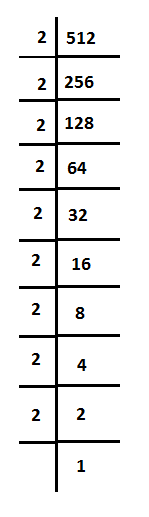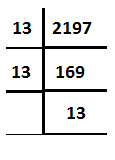# Using Prime Factorisation Find The Cube Roots Of (A) 512 and (B) 2197

The cube root of 512 by prime factorization method512 = (2×2×2) × (2×2×2) × (2×2×2)}

= 8 * 8 * 8

The cube root of 512 is 8.

$$\begin{array}{l} \sqrt{512} = \sqrt{8} \end{array}$$

The cube root of 2197 by prime factorization method2197 = 13 * 13 * 13

The cube root of 2197 is 13

$$\begin{array}{l} \sqrt {2197} = \sqrt{13} \end{array}$$

Explore more such questions and answers at BYJU’S.(5)(0)